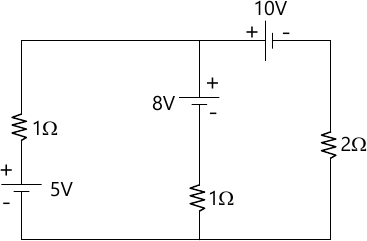MORE IN Basic Electrical Engineering (BEE)
SPPU First Year Engineering (Semester 1)
Basic Electrical Engineering (BEE)
October 2012
Total marks: --
Total time: --
INSTRUCTIONS
(1) Assume appropriate data and state your reasons
(2) Marks are given to the right of every question
(3) Draw neat diagrams wherever necessary

Answer any one question from Q1 & Q2
1 (a) If ?1 and ?2 are RTCs of Material at t1 ?C and t2 ?C respectively, then prove that: [ dfrac {alpha_1}{alpha_2} = 1+ alpha_1 (t_2 ? t_1) ]
6 M
1 (b) If a coil of 150 turns carries a current of 10A and flux linked with it is 0.01 wb, then calculate the inductance of the coil. If the current is uniformly reversed in 0.1 sec., calculate the emf induced. If a second coil of 100 turns is uniformly wound over the first coil, find the mutual inductance between the coils.
6 M

2 (a) Derive an expression for the energy stored per Unit Volume in the Magnetic Field.
6 M
2 (b) Find the current drawn by a crane motor when raising a mass of 1000 kg through a height of 15 meters in 10 sec. The supply is 400V DC, gear efficiency is 0.6 and motor efficiency is 0.8.
6 M

Answer any one question from Q3 & Q4
3 (a) State and derive the expression for the Average Value of Sinusoidally varying alternating quantity.
6 M
3 (b) A 30 kVA, 6000/200V, 50Hz single phase transformer has an iron loss of 500 watt. Its primary and secondary winding resistances are 6 ? and 0.02 ? respectively. Find its efficiency on full load at Vnity p.f.
6 M

4 (a) Derive an expression for energy stored in a Capacitor.
6 M
4 (b) A pure inductance L = 0.1H is connected across voltage of v= 200 sin 314t. Find the rms value of current, instantaneous and average power. Write down expression for current.
6 M

Answer any one question from Q5 & Q6
5 (a) Derive an expression for line current in terms of phase current for three phase delta connected balanced load, with phasor diagram, connected across three phase supply.
6 M
5 (b) A series R-L circuit consist of resistance of 3 ? and inductance of 0.0106H connected across v= 141 sin wt, 60Hz supply. Write down expression for inst. Current drawn by circuit. Calculate average power dissipited and p.f. of the circuit.
7 M

6 (a) Derive an expression for current drawn and power consumed by a circuit consisting of ?R? and ?C? connected in series across v= Vm sin wt supply.
6 M
6 (b) A resistance of 20 ? and coil of inductance 31.8 mH and negligible resistance, are connected in parallel across 230V, 50 Hz supply. Find : (i) currents drawn, (ii) p.f. and (iii) power consumed by the circuit.
7 M

Answer any one question from Q7 & Q8
7 (a) Derive an expression to convert Star Connected Network into its equivalent Delta Connected Network.
6 M
7 (b) Find applying Kirchhoff?s Laws currents in three voltage sources in the network. Shown in fig. 1.7 M

8 (a) State and explain Thevenin?s Theorem.
5 M
8 (b) Apply Superposition Theorem to calculate current flowing in 2? resistance for the network. Shown in fig. 1.8 M

More question papers from Basic Electrical Engineering (BEE)